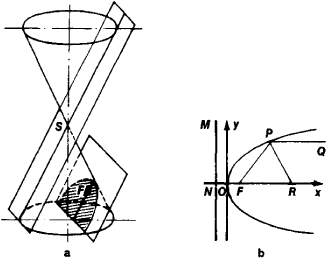# parabola

(redirected from Parabolic Equation)
Also found in: Dictionary, Thesaurus, Acronyms.

## parabola

(pərăb`ələ), plane curve consisting of all points equidistant from a given fixed point (focus) and a given fixed line (directrix). It is the conic sectionconic section
or conic
, curve formed by the intersection of a plane and a right circular cone (conical surface). The ordinary conic sections are the circle, the ellipse, the parabola, and the hyperbola.
cut by a plane parallel to one of the elements of the cone. The axis of a parabola is the line through the focus perpendicular to the directrix. The vertex is the point at which the axis intersects the curve. The latus rectum is the chord through the focus perpendicular to the axis. Examples of this curve are the path of a projectile and the shape of the cross section of a parallel beam reflector.

## parabola

(pă-rab -ŏ-lă) A type of conic section with an eccentricity equal to one. See also paraboloid.

## Parabola

a curve that is the intersection of a circular cone by a plane parallel to a tangent plane to the cone (Figure 1, a); it thus is a conic section. A parabola can also be defined as the locus of points in a plane (Figure 1, b) such that each point is equidistant from a fixed point F of the plane and from a given line MN; F is called the focus and MN the directrix of the parabola. The line passing through the focus perpendicular to the directrix and directed from the directrix toward the focus is the axis of the parabola. The point at which the axis intersects the parabola is the vertex of the parabola.Figure 1

Let us choose the coordinate system xOy, as shown in Figure 1, b. The equation of the parabola then takes the form

y2 = 2px

where p is the length of the segment FN and is called the parameter of the parabola. The parabola is a quadratic curve; it is the graph of the quadratic trinomial y = ax2 + bx + c. It extends to infinity and is symmetric with respect to its axis.

If a light source is placed at the focus of a parabola, the rays reflected by the parabola will form a parallel beam, since the angle formed by the normal PR and the straight line PF connecting any point P of the parabola to the focus is equal to the angle that PR forms with a line parallel to the axis. This property of the parabola is used, for example, in projectors.

## parabola

[pə′rab·ə·lə]
(mathematics)
The plane curve given by an equation of the form y = ax 2+ bx + c.

## parabola

a conic section formed by the intersection of a cone by a plane parallel to its side. Standard equation: y2 = 4ax, where 2a is the distance between focus and directrix
References in periodicals archive ?
Ozbilge, Identification of the unknown diffusion coefficient in a quasi-linear parabolic equation by semigroup approach with mixed boundary conditions, Math.
Ribaud, Cauchy problem for semilinear parabolic equations with initial data in [H.
Continuation backward in time in parabolic equations is a notoriously ill-posed problem with some intriguing applications.
1987) A parabolic equation model for transformation of irregular waves due to refraction, diffraction and breaking.
Matano, Nonincrease of the lap-number of a solution for a one-dimensional semilinear parabolic equation, J.
Hybrid ray optics and parabolic equation methods for radar propagation modeling," International Conference Radar, 58-61, 1992.
Kalantarov, A remark on nonexistence of global solutions to quasi-linear hyperbolic and parabolic equations.
A stable implicit finite difference approximation to a fourth order parabolic equation.
But as far as we know, few works are concerned with the decay estimates of solutions for the p-Laplacian parabolic equation.
The Derrida-Lebowitz-Speer-Spohn (DLSS) equation is a nonlinear fourth order parabolic equation which was proposed to study the interface fluctuations in a spin system , .
Here, we give a simple proof to this well known result via rescaling process which reduces the equation to a random parabolic equation.

Site: Follow: Share:
Open / Close